## Tucker Circles

Let three equal lines,, andbe drawn Antiparallel to the sides of a triangle so that two (sayand) are on the same side of the third line as. Then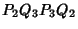is an isosceles Trapezoid, i.e.,,, and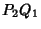are parallel to the respective sides. The Midpoints,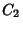, andof the antiparallels are on the respective symmedians and divide them proportionally.

Ifdividesin the same ratio,,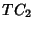,are parallel to the radii,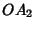, andand equal. Since the antiparallels are perpendicular to the symmedians, they are equal chords of a circle with centerwhich passes through the six given points. This circle is called the Tucker circle.

Ifthen the radius of the Tucker circle iswhere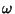is the Brocard Angle.

The Cosine Circle, Lemoine Circle, and Taylor Circle are Tucker circles.